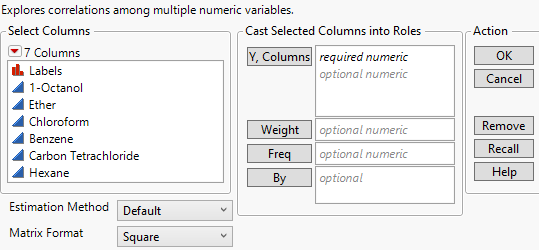Publication date: 11/29/2021

# Launch the Multivariate Platform

Launch the Multivariate platform by selecting Analyze > Multivariate Methods > Multivariate.

Figure 3.3 The Multivariate Launch WindowY, Columns

Identifies one or more response columns. The response columns must have a numeric data type, but the modeling type can be continuous or ordinal.

Note: If you specify an ordinal response variable, a JMP alert appears after you click OK in the launch window. The alert indicates which variables are ordinal and confirms that you intended to include the ordinal variables in the analysis.

Weight

Identifies one column whose numeric values assign a weight to each row in the analysis.

Freq

Identifies one column whose numeric values assign a frequency to each row in the analysis.

By

Produces a separate report of each level of By variable. If more than one By variable is assigned, a separate report is produced for each possible combination of the levels of the By variables.

Estimation Method

Specifies the method for calculating the correlations. REML and Pairwise are the methods used most frequently. Several of these methods address the treatment of missing data. You can also estimate missing values by using a method other than Row-wise and then selecting the Impute Missing Data command. See Impute Missing Data.

Default

The Default option uses either the Row-wise, Pairwise, or REML methods.

Row-wise estimation is used for data tables with no missing values.

Pairwise estimation is used for data tables with missing values and either more than 10 columns, more than 5,000 rows, or more columns than rows.

REML estimation is used otherwise.

Note: When the Default option would otherwise result in REML estimation, but the fit does not converge properly, the platform reverts to the Pairwise method. This can happen when there are missing values in your data table and at least one of the following situations applies: if your data table has fewer than 10 columns, fewer than 5,000 rows, or fewer columns than rows. If the estimation method shown is Pairwise, this means that the REML fit did not converge.

REML

Restricted maximum likelihood (REML) estimation uses all of the data, even if missing values are present. Due to a bias-correction factor, this method is slow if the data set is large and there are many missing values. Therefore, REML is most useful for smaller data sets. If there are no missing cells in the data, then the REML and ML estimates are equivalent and equal to the sample covariance matrix. If there are missing cells, REML’s variance and covariance estimates are less biased than the estimates from ML estimation. For statistical details, see REML.

ML

Maximum likelihood (ML) estimation uses all of the data, even if missing values are present. Because the estimates from ML are generated quickly, this method is most useful for large data tables with missing data.

Robust

Robust estimation uses all of the data, even if missing values are present. This method down-weights extreme values and is therefore useful for data tables that might have outliers. For statistical details, see Robust.

Row-wise

Row-wise estimation calculates the Pearson correlation for each pair of columns. For statistical details, see Pearson Product-Moment Correlation. Row-wise estimation does not use rows with missing values. This method is useful for excluding observations with missing data.

Pair-wise

Pair-wise estimation uses all of the data, even if missing values are present. This estimation method calculates Pearson correlations for each pair of columns using all observations with nonmissing values for those two columns. For statistical details, see Pearson Product-Moment Correlation. Pair-wise estimation is most useful when a data table has missing values and either more columns than rows, more than 10 columns, or more than 5,000 rows.

Note: If you select REML, ML, or Robust and your data table contains more columns than rows and has missing values, JMP switches the Estimation Method to Pairwise.

Matrix Format

Select a format option for the Scatterplot Matrix. The Square option displays plots for all ordered combinations of columns. Lower Triangular displays plots below the diagonal, with the first n - 1 columns on the horizontal axis. Upper Triangular displays plots above the diagonal, with the first n - 1 columns on the vertical axis.